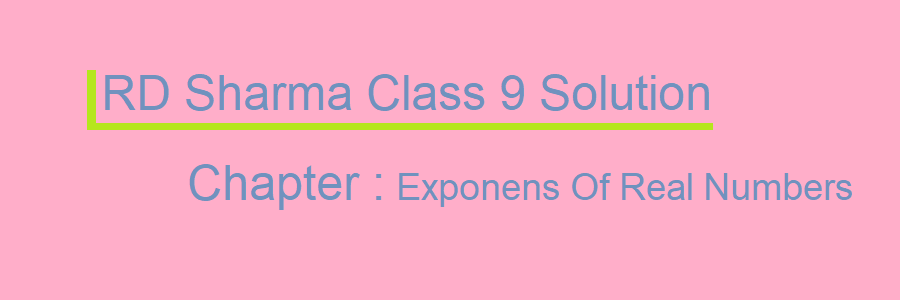# RD Sharma Class 9 Solution Chapter 2 Exponents Of Real Numbers

## RD Sharma Maths Solutions for Class 9 Chapter 2: Exponents of Real Numbers

In chapter 2 of RD Sharma’s textbook, the problems related to Exponents of Real numbers are solved in a simple way. The RD Sharma Solution for class 9 also has solved examples of some important topics such as Laws of Integral Exponents, Integral Exponents of a Real Number, and Rational Exponents of a Real Number. As per the latest syllabus, the topics of Exponents of Real Numbers are prepared by subject matter experts. The solution also aims to ease the final examinations of the students by answering the problems in this chapter. The RD Sharma Solution for Exponents of Real Numbers primarily explains problems of Product with the same bases, Quotients with the same bases, Power raised to a power, Quotients to a power, Zero power, Product to a power, Fractional Exponent Rule, and Negative Exponent Rule. Practicing these problems is extremely important for students to solve questions on the final exams. The exercises that explain essential topics like Exponents and Laws of Exponents are given below with the links. Students can click on the links to access RD Sharma Maths Solutions for Class 9 Chapter 2 Exponents of Real Numbers.Class 9 Maths Ch 2

For more update follow rd sharma solution page

Updated: July 25, 2022 — 2:39 pm